# CBSE Class 10 Maths Chapter 10 - Circles Objective Questions

The CBSE Class 10 Maths Chapter 10 Circles Objective Questions is based on concepts like the properties of circle and the existence of  the tangents to a circle. In this chapter, taken from the Unit 4 Geometry of CBSE Class 10 Maths Syllabus 2021, the students are introduced to some complex terms such as tangents, tangents to a circle, number of tangents from a point on the circle and so on. Also, keeping in mind the fact that the number of MCQs is likely to increase for the upcoming board exams, we have compiled here the objective questions from CBSE Class 10 Maths Chapter 10-Circles.

These CBSE Class 10 Maths objective questions are most likely categorised topic wise.

### Sub-Topics Discussed in Chapter 10- Circles

Find here some of the sub-topics that are discussed via these objective questions we have given below:

• 10.1 Introduction to Circles (5 MCQs From This Topic)
• 10.2 Tangent to the Circle (8 MCQs From The Given Topic)
• 10.3 Theorems (7 MCQs Listed From Topic)

## Download CBSE Class 10 Maths Chapter 10-Circles Objective Questions PDF Free

### Introduction to Circles

1. Two concentric circles of radii a and b (a > b) are given. Find the length of the chord of the larger circle which touches the smaller circle.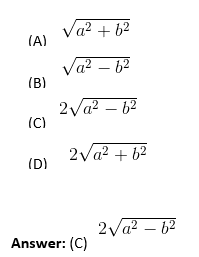Solution: Let O be the common center of the two circles and AB be the chord of the larger circle which touches the smaller circle at C.

Join OA and OC.

Then OC ⊥ AB

Let OA = a and OC = b.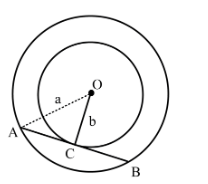Since OC ⊥ AB, OC bisects AB

[∵ perpendicular from the centre to a chord bisects the chord].

In right Δ ACO, we have

OA2=OC2+AC2    [by Pythagoras’ theorem]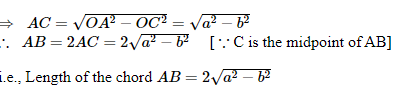1. Three circles touch each other externally. The distance between their centres is 5 cm, 6 cm and 7 cm. Find the radii of the circles.
1. 2 cm, 3 cm, 4 cm
2. 1 cm, 2 cm, 4 cm
3. 1 cm, 2.5 cm, 3.5 cm
4. 3 cm, 4 cm, 1 cm

Answer: (A) 2 cm, 3 cm, 4 cm

Solution: Consider the below figure wherein three circles touch each other externally.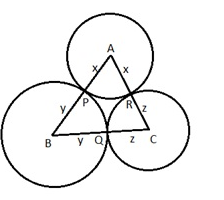Since the distances between the centres of these circles are 5 cm, 6 cm and 7 cm respectively, we have the following set of equations with respect to the above diagram:

x+y = 5     …..(1)

y+z = 6     …… (2) (⇒ y=6-z)…   (2.1)

x+z = 7     …..(3)

Adding (1), (2) and (3), we have 2(x+y+z) =5+6+7=18

⟹x+y+z=9…. (4)

Using (1) in (4), we have 5+z=9⟹z=4

Now using, (3) ⟹x=7−z=7−4=3

And (2.1) ⟹y=6−z=6−4=2

Therefore, the radii of the circles are 3 cm, 2 cm and 4 cm.

1. A point P is 13 cm from the centre of the circle. The length of the tangent drawn from P to the circle is 12cm. Find the radius of the circle.
1. 5cm
2. 7cm
3. 10cm
4. 12cm

Solution: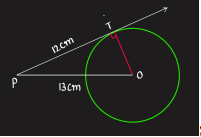Since,

tangent to  a circle is perpendicular to the radius through the point of contact

So, ∠OTP=90°

So, in triangle OTP

(OP)2=(OT)2+(PT)2

132=(OT)2+122

(OT)2=132−122

OT2=25

OT= √25

OT=5

So, radius of the circle is 5 cm

1. In the adjoining figure ‘O’ is the center of circle, ∠CAO = 25°  and ∠CBO = 35°. What is the value of ∠AOB?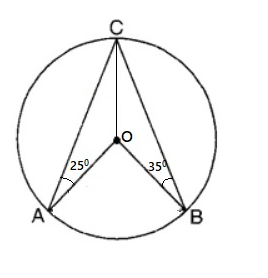1. 120°
2. 110°
3. 55°
4. Data insufficient

Solution: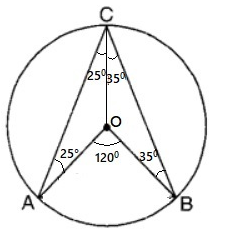In ΔAOC,

OA=OC      ——–(radii of the same circle)

∴ΔAOC is an isosceles triangle

→∠OAC=∠OCA=25°—– (base angles of an isosceles triangle )

In ΔBOC,

OB=OC      ——–(radii of the same circle)

∴ΔBOC is an isosceles triangle

→∠OBC=∠OCB=35° —–(base angles of an isosceles triangle )

∠ACB=25°+35°=60°

∠AOB=2×∠ACB —-(angle at the center is twice the angle at the circumference)

= 2×60°

=120°

1. A: What is a line called, if it meets the circle at only one point?

B: Collection of all points equidistant from a fixed point is ______.

1: Chord

2: Tangent

3: Circle

4: Curve

5: Secant

Which is correct matching?

1. A-2; B-4
2. A-5; B-4
3. A-4; B-1
4. A-2; B-3

Solution: Tangent is a line which touches the circle at only 1 point.

Collection of all points equidistant from a fixed point is called a circle.

### Tangent to the Circle

1. A point A is 26 cm away from the centre of a circle and the length of tangent drawn from A to the circle is 24 cm. Find the radius of the circle.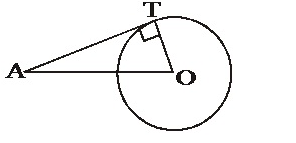1. 2√313
2. 12
3. 7
4. 10

Solution: Let O be the centre of the circle and let A be a point outside the circle such that OA = 26 cm.

Let AT be the tangent to the circle.

Then, AT = 24 cm. Join OT.

Since the radius through the point of contact is perpendicular to the tangent, we have ∠OTA = 90°. In right △ OTA, we have

OT2 = OA2 –  AT2

= [(26)2 – (24)2] = (26 + 24) (26 – 24) = 100.

=>       OT = √100 = 10cm

Hence, the radius of the circle is 10 cm.

1. The quadrilateral formed by joining the angle bisectors of a cyclic quadrilateral is a
2. parallelogram
3. square
4. Rectangle

Solution: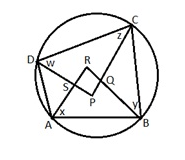ABCD is a cyclic quadrilateral ∴∠A +∠C = 180° and ∠B+ ∠D =  180°

1/2∠A+1/2 ∠C = 90° and 1/2 ∠B+1/2 ∠D =  90°

x + z = 90° and y + w =  90°

In △ARB and △CPD, x+y + ∠ARB = 180° and z+w+ ∠CPD =  180°

∠ARB = 180° – (x+y) and ∠CPD = 180° – (z+w)

∠ARB+∠CPD = 360° – (x+y+z+w) = 360° – (90+90)

= 360° – 180° ∠ARB+∠CPD =  180°

∠SRQ+∠QPS = 180°

The sum of a pair of opposite angles of a quadrilateral PQRS is 180∘.

1. In the given figure, AB is the diameter of the circle. Find the value of ∠ ACD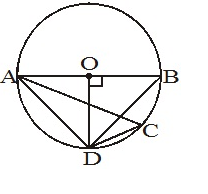1. 25°
2. 45°
3. 60°
4. 30°

∠ ODB = ∠ OBD

∠ ODB + ∠OBD + ∠BOD = 180°

2∠ODB + 90° = 180°

∠ODB = 45°

∠OBD = ∠ACD (Angle subtended by the common chord AD)

Therefore ∠ACD = 45°

1. Find the value of ∠ DCE: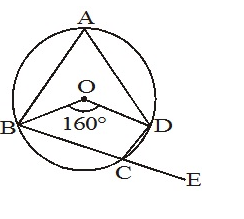1. 80°
2. 75°
3. 90°
4. 100°

∠ BAD + ∠ BCD = 180°

∠ BCD = 100°

∠ DCE = 180°- ∠ BCD

∠ DCE = 180°– 100°

∠ DCE = 80°

1. ABCD is a cyclic quadrilateral PQ is a tangent at B. If ∠DBQ = 65°, then ∠BCD is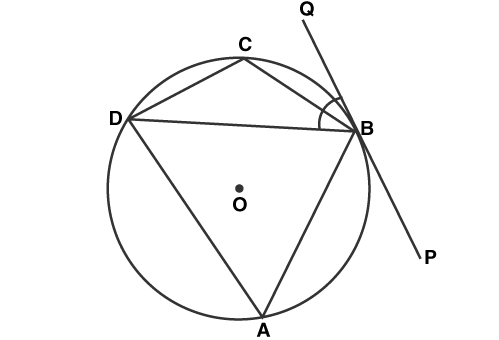1. 35°
2. 85°
3. 90°
4. 115°

Solution: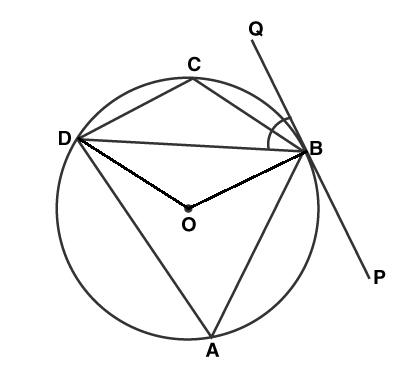Join OB and OD

We know that OB is perpendicular to PQ

∠OBD = ∠OBQ – ∠DBQ

∠OBD = 90° – 65°

∠OBD = 25°

∠OBD = ∠ODB = 25°

In △ODB

∠OBD + ∠ODB + ∠BOD = 180°

25° + 25° + ∠BOD = 180°

∠BOD = 130°

(Angle subtended by a chord on the centre is double the angle subtended on the circle)

∠BCD + 65° = 180°

∠BCD = 115°

1. In a circle of radius 5 cm, AB and AC are the two chords such that AB = AC = 6 cm. Find the length of the chord BC
1. None of these
2. 9.6cm
3. 10.8cm
4. 4.8cm

Solution: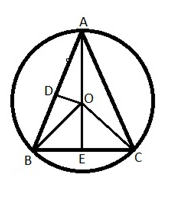Consider the triangles OAB and OAC are congruent as

AB=AC

OA is common

OB = OC = 5cm.

So ∠OAB = ∠OAC

Draw OD perpendicular to AB

Hence AD = AB/2 = 6/2 = 3 cm as the perpendicular to the chord from the center bisects the chord.

OD2 = 52 – 32

OD = 4 cm

So Area of OAB = 1/2 AB x OD = 1/2 6 x 4 = 12 sq. cm.        ….. (i)

Now AO extended should meet the chord at E and it is middle of the BC as ABC is an isosceles with AB= AC

Triangles AEB and AEC are congruent as

AB =AC

AE common,

∠OAB = ∠ OAC.

Therefore triangles being congruent, ∠AEB = ∠AEC = 90°

Therefore BE is the altitude of the triangle OAB with AO as base.

Also this implies BE =EC or BC =2BE

Therefore the area of the △ OAB

= ½×AO×BE = ½ × 5×BE = 12 sq. cm as arrived in eq (i).

BE = 12 × 2/5 = 4.8cm

Therefore BC = 2BE = 2×4.8 cm = 9.6 cm.

1.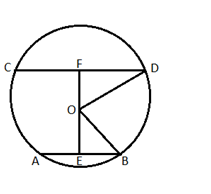In a circle of radius 17 cm, two parallel chords are drawn on opposite sides of a diameter. The distance between the chords is 23 cm. If the length of one chord is 16 cm, then the length of the other is

1. None of these
2. 15cm
3. 30cm
4. 23cm

Solution: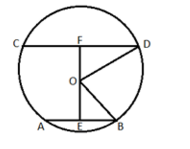Given that

OB = OD =17

AB = 16 ⇒ AE = BE = 8 cm as perpendicular from centre to the chord bisects the chords

EF = 23 cm

Consider   △OEB

OE2 =  OB2 – EB2

OE2 =  172 –  82

OE = 15 CM

OF = EF – OE

OF = 23 – 15

OF = 8 cm

FD2 = OD2 – OF2

FD2 = 172 –  82

FD = 15

Therefore CD = 2FD = 30 cm

1. The distance between the centres of equal circles each of radius 3 cm is 10 cm. The length of a transverse tangent AB is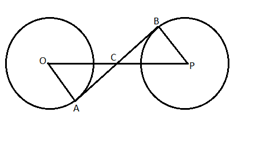1. 10cm
2. 8cm
3. 6cm
4. 4cm

Solution: ∠OAC = ∠CBP = 90°

∠OCA = ∠PCB (Vertically opposite angle)

Triangle OAC is similar to PBC

OA/PB = OC/PC

3/3 = OC/PC

OC = PC

But PO = 10 cm

Therefore OC = PC = 5cm

AC= OC2 –  OA2

AC= 52 –  32

AC = 4 cm

Similarly BC = 4 cm

Therefore AB = 8 cm

### Theorems

1. A point P is 10 cm from the center of a circle. The length of the tangent drawn from P to the circle is 8 cm. The radius of the circle is equal to
1. 4cm
2. 5cm
3. None of these
4. 6cm

Solution: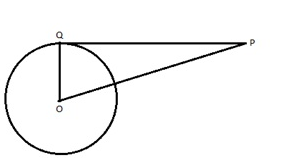Given that OP = 10 cm, PQ = 8 cm

As, tangent to a circle is perpendicular to the line joining the centre of the circle to the tangent at the point of contact to the circle.

Angle OQP = 902

Applying Pythagoras theorem to triangle OPQ

OQ2 + QP2 = OP2

OQ+ 8= 102

OQ= 100-64

=36

OQ = 6 cm.

Ans: Radius of the circle is 6 cm.

1. In fig, O is the centre of the circle, CA is tangent at A and CB is tangent at B drawn to the circle. If ∠ACB = 75°, then ∠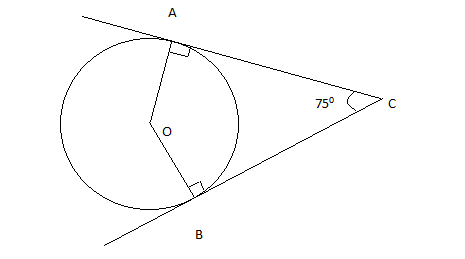AOB=

1. 75°
2. 85°
3. 95°
4. 105°

Solution: ∠OAC = ∠OBC = 90°​

∠OAC + ∠OBC + ∠ACB + ∠AOB = 360°​  ….. (sum of angles of a quadrilateral)

90°​​ + 90°​​ + 75°​​ + ∠AOB = 360°

∠AOB = 105°

1. PA and PB are the two tangents drawn to the circle. O is the centre of the circle. A and B are the points of contact of the tangents PA and PB with the circle. If ∠OPA = 35°, then ∠POB =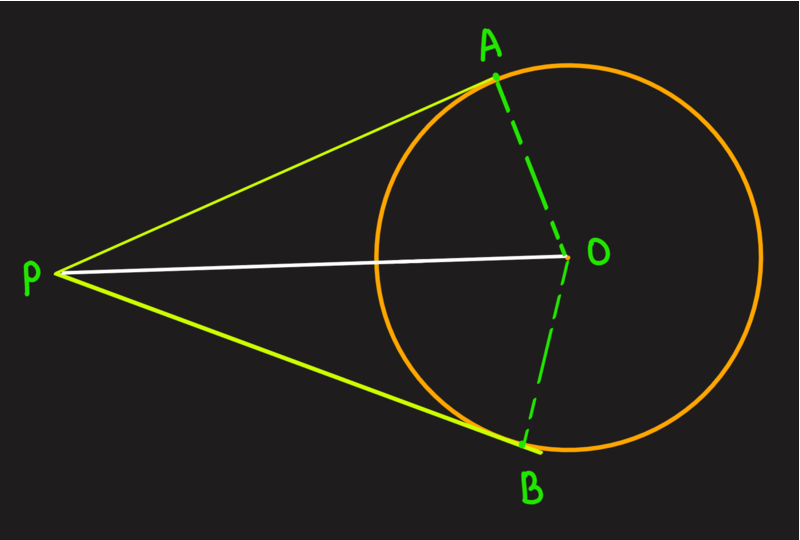1. 55°
2. 65°
3. 85°
4. 75°

Solution: ∠OAP =∠OBP = 90°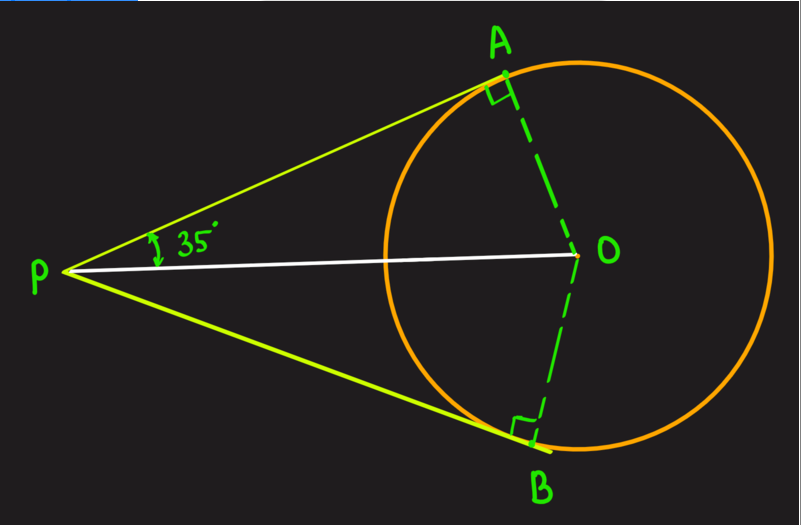∠AOP = 180°- 35°- 90°

∠AOP = 55°

OA = OB

AP = PB

OP is common base

Therefore △OAP ≅ △OBP

∠AOP = ∠BOP

Ans: ∠BOP = 55°

1. A tangent PQ at a point P of a circle of radius 5 cm meets a line through the center O at a point Q such that OQ =13  cm. Length PQ is:
1. √119
2. 8.5cm
3. 13cm
4. 12cm

Solution: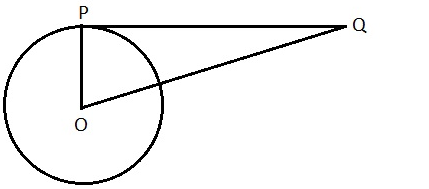Given that OP = 5 cm, OQ = 13 cm

To find PQ

Applying Pythagoras theorem to triangle OPQ

OP2 + QP2 = OQ2

5+ QP2 = 132

QP2 = 169 – 25= 144

QP = √144cm

QP =12 cm

1. The length of the tangent from a point A to a circle, of radius 3 cm, is 4 cm. The distance of A from the centre of the circle is
1. √7
2. 7cm
3. 5cm
4. 25cm

Solution: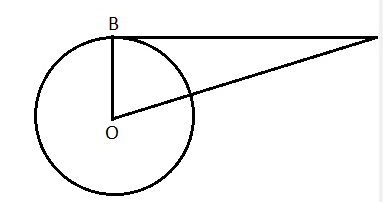Given that AB = 4 cm, OB =3 cm

To find OA

Applying Pythagoras theorem to triangle OAB

OB+ AB2 = OA2

32 + 4= OA

OA= 25

OA = 5 cm

Therefore the distance of A from the centre of the circle is 5 cm.

1. If TP and TQ are two tangents to a circle with center O such that ∠POQ = 110°, then, ∠PTQ is equal to: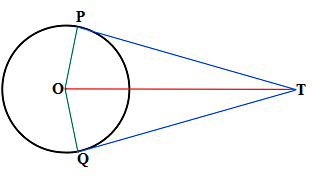1. 90°
2. 80°
3. 70°
4. 60°

Solution: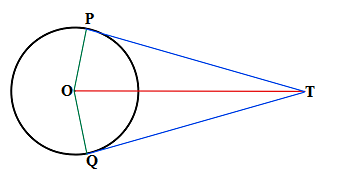We know that ∠OQT=∠OPT=90°.

Also ∠OQT+∠OPT+∠POQ+∠PTQ=360°.

∠PTQ=360°–90°–90°–110°

= 70°

∴∠PTQ=70°

1. In the given figure, PAQ is the tangent. BC is the diameter of the circle. ∠BAQ = 60°, find  ∠ABC :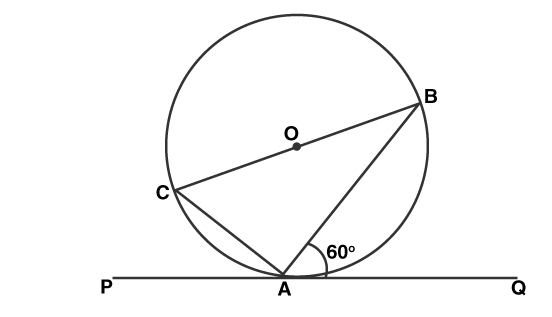1. 25°
2. 30°
3. 45°
4. 60°

Solution: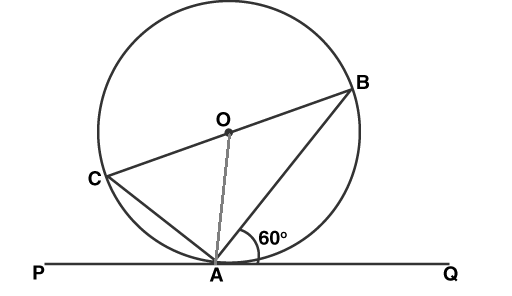Join OA

As the tangent at any point of a circle is perpendicular to the radius through the point of contact

∠OAQ = 90°

∠OAB = ∠OAQ – ∠BAQ

∠OAB = 90° – 60°

∠OAB = 30°

∠OAB = ∠OBA

Therefore ∠OBA = 30°

∠ABC = 30°

These objective questions from the chapter 10 of CBSE Class 10 Textbooks are framed based on the concepts involved in circles. This will help the students to understand the concept better and also for them to score good marks in the examination.

Find also some extra questions for the students to solve.

### CBSE Class 10 Maths Chapter 10 Extra Questions

1. What is the number of tangents drawn at a point of the circle?
(a) one
(b) two
(c) none
(d) infinite

2. If  the length of a tangent drawn from a point at a distance of 10 cm of circle is 8 cm. The, what is radius of the circle?
(a) 4 cm
(b) 5 cm
(c) 6 cm
(d) 7 cm

3. What is the distance between two parallel tangents of acircle of radius 4 cm?
(a) 2 cm
(b) 4 cm
(c) 6 cm
(d) 8 cm